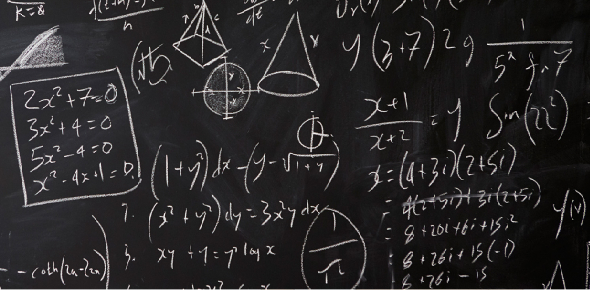# Polynomial Equations

4 Questions | Total Attempts: 35SettingsQuiz to assess knowledge of the history and development of polynomial equations and their possible solutions.

Related Topics
• 1.
The Fundamental Theorem of Algebra states that every polynomial equation of degree n has ? solution(s).
• A.

"at most n"

• B.

"exactly n"

• C.

1

• D.

2

• E.

"more than n"

• 2.
Who discovered the Quintic Formula?
• A.

Abel

• B.

Galois

• C.

Ruffini

• D.

Einstein

• E.

None of these

• 3.
What major conclusion resulted from the work of Ruffini, Abel, and Galois?
• 4.
Solutions to polynomial equations can be ? or ?.